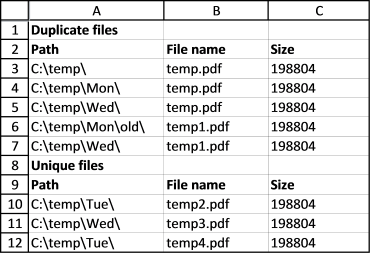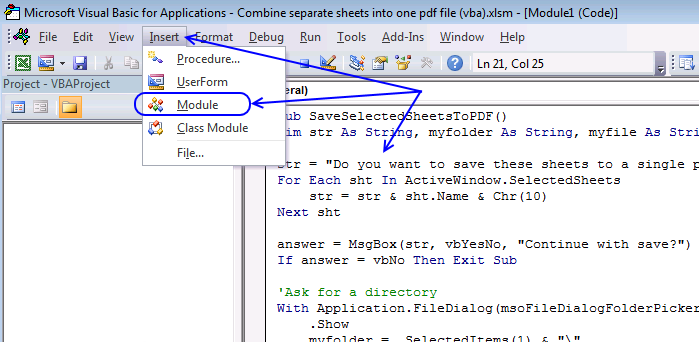Author: Oscar Cronquist Article last updated on April 15, 2021This is a follow up to my last post Compare file names in two different folder locations and their subfolders, the obvious question after reading that article is "How do I find duplicate files in a single folder and its subfolders?"

This article answers that question, there are two macros below: "FindDuplicateFiles" and "Recursive". These macros are perhaps more useful for my blog readers than the macro in my last post.

I imagine the macros would be great for finding duplicate mp3 or picture files. Note, they compare only the file names, not the file sizes.

## 1. VBA Code

Check article Compare file names in two different folder locations and their sub folders where I explain the VBA code.

```Sub FindDuplicateFiles()
Dim pth1 As String
Dim arrd() As Variant
Dim arru() As Variant
ReDim arrd(0 To 2, 0)
ReDim arru(0 To 2, 0)

With Application.FileDialog(msoFileDialogFolderPicker)
.Show
pth1 = .SelectedItems(1) & "\"
End With

Set x = ActiveSheet

Application.ScreenUpdating = False
x.Range("A1") = "Duplicate files"
x.Range("A2") = "Path"
x.Range("B2") = "File name"
x.Range("C2") = "Size"
x.Range("A:F").Font.Bold = False
x.Range("A1:C2").Font.Bold = True

Recursive pth1

Lrow = ActiveSheet.Range("A" & Rows.Count).End(xlUp).Row
x.Range("A2:C" & Lrow).Sort Key1:=x.Range("B1"), Header:=xlYes

arr1 = x.Range("A3:C" & Lrow).Value

x.Range("A3:C" & Lrow).Clear

For r1 = LBound(arr1, 1) + 1 To UBound(arr1, 1)

If arr1(r1, 2) = arr1(r1 - 1, 2) Then

arrd(0, UBound(arrd, 2)) = arr1(r1 - 1, 1)
arrd(1, UBound(arrd, 2)) = arr1(r1 - 1, 2)
arrd(2, UBound(arrd, 2)) = arr1(r1 - 1, 3)

ReDim Preserve arrd(UBound(arrd, 1), UBound(arrd, 2) + 1)

arr1(r1 - 1, 1) = ""
arr1(r1 - 1, 2) = ""
arr1(r1 - 1, 3) = ""

chk = True

Else

If chk = True Then

arrd(0, UBound(arrd, 2)) = arr1(r1 - 1, 1)
arrd(1, UBound(arrd, 2)) = arr1(r1 - 1, 2)
arrd(2, UBound(arrd, 2)) = arr1(r1 - 1, 3)
chk = False

ReDim Preserve arrd(UBound(arrd, 1), UBound(arrd, 2) + 1)

arr1(r1 - 1, 1) = ""
arr1(r1 - 1, 2) = ""
arr1(r1 - 1, 3) = ""

Else

arru(0, UBound(arru, 2)) = arr1(r1 - 1, 1)
arru(1, UBound(arru, 2)) = arr1(r1 - 1, 2)
arru(2, UBound(arru, 2)) = arr1(r1 - 1, 3)

ReDim Preserve arru(UBound(arru, 1), UBound(arru, 2) + 1)

arr1(r1 - 1, 1) = ""
arr1(r1 - 1, 2) = ""
arr1(r1 - 1, 3) = ""

End If

End If

Next r1

If chk = True Then
arrd(0, UBound(arrd, 2)) = arr1(r1 - 1, 1)
arrd(1, UBound(arrd, 2)) = arr1(r1 - 1, 2)
arrd(2, UBound(arrd, 2)) = arr1(r1 - 1, 3)
Else
arru(0, UBound(arru, 2)) = arr1(r1 - 1, 1)
arru(1, UBound(arru, 2)) = arr1(r1 - 1, 2)
arru(2, UBound(arru, 2)) = arr1(r1 - 1, 3)
End If

x.Range("A3").Resize(UBound(arrd, 2) + 1, UBound(arrd, 1) + 1) = Application.Transpose(arrd)

x.Range("A" & UBound(arrd, 2) + 3) = "Unique files"
x.Range("A" & UBound(arrd, 2) + 4) = "Path"
x.Range("B" & UBound(arrd, 2) + 4) = "File name"
x.Range("C" & UBound(arrd, 2) + 4) = "Size"
x.Range("A" & UBound(arrd, 2) + 3 & ":C" & UBound(arrd, 2) + 4).Font.Bold = True
x.Range("A" & UBound(arrd, 2) + 5).Resize(UBound(arru, 2) + 1, UBound(arru, 1) + 1) = Application.Transpose(arru)

x.Columns("A:C").AutoFit

End Sub

Sub Recursive(FolderPath As String)
Dim Value As String, Folders() As String
Dim Folder As Variant, a As Long
ReDim Folders(0)
If Right(FolderPath, 2) = "\\" Then Exit Sub
Value = Dir(FolderPath, &H1F)
Do Until Value = ""
If Value = "." Or Value = ".." Then
Else
If GetAttr(FolderPath & Value) = 16 Or GetAttr(FolderPath & Value) = 48 Then
Folders(UBound(Folders)) = Value
ReDim Preserve Folders(UBound(Folders) + 1)
Else
Lrow = ActiveSheet.Range("A" & Rows.Count).End(xlUp).Row + 1
ActiveSheet.Range("A" & Lrow) = FolderPath
ActiveSheet.Range("B" & Lrow) = Value
ActiveSheet.Range("C" & Lrow) = FileLen(FolderPath & Value)
End If
End If
Value = Dir
Loop
For Each Folder In Folders
Recursive FolderPath & Folder & "\"
Next Folder
End Sub

```

## 2. How do I use this macro?The easiest way to go is to get the excel file at the bottom of this post and run it from there. But if you want to copy the macro to your workbook, follow these steps:

1. Copy the code above.
2. Go to the VB Editor (Alt+F11).
3. Press with left mouse button on "Insert" on the menu.
4. Press with left mouse button on "Module".
5. Paste code to code window.

## 3. How do I start the macro?

1. Go to "Developer" tab on the ribbon.
2. Press with left mouse button on "Macros" button.
3. Select macro name "FindDuplicateFiles".
4. Press with left mouse button on "Run" button.

## 4. What happens when I run the macro?

1. Select a folder on your harddrive or network drive.
2. A new sheet is inserted.
3. Sheet is populated with data.
4. Macro ends.